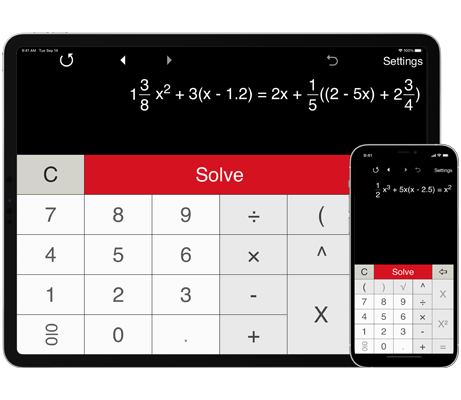Solves linear and quadratic equations with fractions.
Stores history.

## Solving quadratic equations with fractions step by step

Need help solving quadratic equations involving fractions? Use a quadratic equations calculator to solve quadratic equations with fractional coefficients.

Quadratic equations calculator provides three pre-defined formats for solving quadratic equations in the form
ax2 + bx + c = 0, ax2 + bx = 0 and ax2 + c = 0.